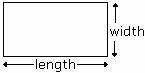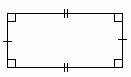Definition Of Rectangle

A rectangle is a quadrilateral with four right angles.

The either sides of a rectangle are parallel and of equal length.
All squares are rectangles.
Perimeter of a rectangle is give as 2(length + width).
Area of a rectangle = length × widthExample of RectangleThe four angles are right angles in a rectangle. The opposite sides are parallel and are congruent.

Solved Example on Rectangle

Ques: A corn field is in the form of a rectangle with length 14 yards and width 11 yards. Find the area of the field.

Choices:

A. 121 square yards
B. 196 square yards
C. 154 square yards
D. 616 square yards